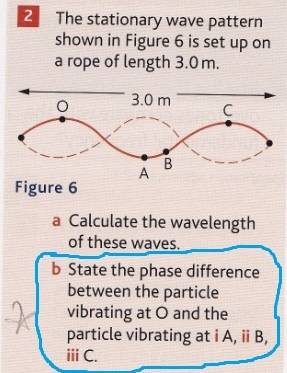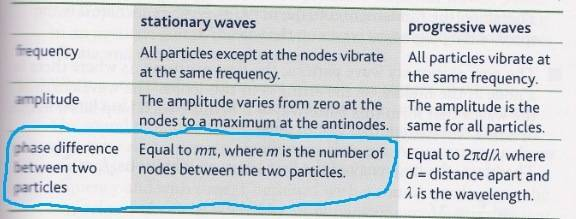# Waves and phase difference

• question dude
B ii) and 360 degrees for part B iii). My mistake was thinking that the answer for part B ii) was 1 * (pi) = (pi) which is incorrect, the answer is actually 180 degrees.I see your point, and I can sort of understand why particle C is at 0 degrees relative to particle O. But what's up with the explanation and equations given in my textbook?

## Homework StatementIts part B ii) and iii) that I'm stuck on.

## Homework Equations

Apparently for stationary waves, the phase difference between two particles = m(pi), where m is the number of nodes between the particles. This is according to my textbook here:## The Attempt at a Solution

So following that info above from my textbook, for B ii), it should be 1 * (pi) = (pi) or 180 in degrees, 1 * (pi) follows from there being only one node between particles O and B. But I got it wrong, the answer at the back is 225 degrees.

and for B iii), I'm even more confused, I done: 2 * (pi), because there's two nodes between particles O and C. So I had the answer as 2(pi) or 360 degrees. But I'm wrong, the answers at the back has it as 0

so what's going on here?question dude said:

## Homework StatementIts part B ii) and iii) that I'm stuck on.

## Homework Equations

Apparently for stationary waves, the phase difference between two particles = m(pi), where m is the number of nodes between the particles. This is according to my textbook here:## The Attempt at a Solution

So following that info above from my textbook, for B ii), it should be 1 * (pi) = (pi) or 180 in degrees, 1 * (pi) follows from there being only one node between particles O and B. But I got it wrong, the answer at the back is 225 degrees.

and for B iii), I'm even more confused, I done: 2 * (pi), because there's two nodes between particles O and C. So I had the answer as 2(pi) or 360 degrees. But I'm wrong, the answers at the back has it as 0

so what's going on here?Firstly part 3.

You stand beside someone - each facing North - and you are about to each start rotating on the spot. The angle between the directions you are facing will be your phase difference.
Now you start first, and your friend will start rotating soon. You actually rotate through 360 degrees before your friend joins in the spinning.
What will be the angle between the directions you are both facing when you are both rotating [at the same speed]?

question dude said:

## Homework StatementIts part B ii) and iii) that I'm stuck on.

## Homework Equations

Apparently for stationary waves, the phase difference between two particles = m(pi), where m is the number of nodes between the particles. This is according to my textbook here:## The Attempt at a Solution

So following that info above from my textbook, for B ii), it should be 1 * (pi) = (pi) or 180 in degrees, 1 * (pi) follows from there being only one node between particles O and B. But I got it wrong, the answer at the back is 225 degrees.

and for B iii), I'm even more confused, I done: 2 * (pi), because there's two nodes between particles O and C. So I had the answer as 2(pi) or 360 degrees. But I'm wrong, the answers at the back has it as 0

so what's going on here?I am assuming you got B (i) correct

Compare points A and B of the wave.

When A is at its lowest point, how would you describe the position of B?
When A is at its mean position, how would you describe the position of B?
When A is at its highest point, how would you describe the position of B?

Edit: Just re-read your post, and the answer in the back of the book (225) is wrong - never happens for a standing wave - your answer was correct.

PeterO said:
Firstly part 3.

You stand beside someone - each facing North - and you are about to each start rotating on the spot. The angle between the directions you are facing will be your phase difference.
Now you start first, and your friend will start rotating soon. You actually rotate through 360 degrees before your friend joins in the spinning.
What will be the angle between the directions you are both facing when you are both rotating [at the same speed]?

0 degrees.

I see your point, and I can sort of understand why particle C is at 0 degrees relative to particle O. But what's up with the explanation and equations given in my textbook?

phase difference = m(pi), is this wrong?

PeterO said:
I am assuming you got B (i) correct

Compare points A and B of the wave.

When A is at its lowest point, how would you describe the position of B?
When A is at its mean position, how would you describe the position of B?
When A is at its highest point, how would you describe the position of B?

Yeah I got part B i) as 180 degrees which is the same as the answers at the back, I used the m(pi) equation, and just did 1 * (pi), because there's only one node between A and O

1) When A is at its lowest point, how would you describe the position of B?
2) When A is at its mean position, how would you describe the position of B?
3) When A is at its highest point, how would you describe the position of B?

1) same as shown on the diagram
2) B would be in mean position as well. When A is in mean position, that curved part where its sitting on in the diagram would become straight (horizontal)
3) B would be just below A

Edit: Just re-read your post, and the answer in the back of the book (225) is wrong - never happens for a standing wave - your answer was correct

errr I'm confused

question dude said:
0 degrees.

I see your point, and I can sort of understand why particle C is at 0 degrees relative to particle O. But what's up with the explanation and equations given in my textbook?

phase difference = m(pi), is this wrong?

Yeah I got part B i) as 180 degrees which is the same as the answers at the back, I used the m(pi) equation, and just did 1 * (pi), because there's only one node between A and O

1) When A is at its lowest point, how would you describe the position of B?
2) When A is at its mean position, how would you describe the position of B?
3) When A is at its highest point, how would you describe the position of B?

1) same as shown on the diagram
2) B would be in mean position as well. When A is in mean position, that curved part where its sitting on in the diagram would become straight (horizontal)
3) B would be just below A

I was kind of hoping for:

1) When A is at its lowest point , B is at its lowest point.
2) When A is at its mean position, B is at its mean position.
3) When A is at its highest point, B is at its highest point.

in other words, B is in phase with A, so if A is 180 degrees of of phase, so is B.

With standing waves, when comparing any two points, they are either In phase, or 180 degrees out of phase - there are no other comparisons.

When looking at the original diagram, every point on the loop that includes O is about to move down. Every point on the loop including A & B is about to move up. Every point on the loop including C is about to move down [in phase with O!].

The phase difference of two points on any wave will never be more than 360 degrees out of phase - in fact 360 degrees out of phase is in phase.

So is you slavishly use that m(π) formula, you have to repeatedly subtract 2π until the answer is less than 2π.

PeterO said:
I was kind of hoping for:

1) When A is at its lowest point , B is at its lowest point.
2) When A is at its mean position, B is at its mean position.
3) When A is at its highest point, B is at its highest point.

in other words, B is in phase with A, so if A is 180 degrees of of phase, so is B.

With standing waves, when comparing any two points, they are either In phase, or 180 degrees out of phase - there are no other comparisons.

When looking at the original diagram, every point on the loop that includes O is about to move down. Every point on the loop including A & B is about to move up. Every point on the loop including C is about to move down [in phase with O!].

The phase difference of two points on any wave will never be more than 360 degrees out of phase - in fact 360 degrees out of phase is in phase.

So is you slavishly use that m(π) formula, you have to repeatedly subtract 2π until the answer is less than 2π.

yeah that makes sense, but what is the point of that m(pi) formula? seems a bit pointless, even misleading to me

and also, what if I get asked to compare a particle on a loop and a particle on the exact point of the node?

the particle on the node would constantly be stationary while the other particle goes up and down, so how would you describe the phase difference between them? is it even possible?

question dude said:
yeah that makes sense, but what is the point of that m(pi) formula? seems a bit pointless, even misleading to me

and also, what if I get asked to compare a particle on a loop and a particle on the exact point of the node?

the particle on the node would constantly be stationary while the other particle goes up and down, so how would you describe the phase difference between them? is it even possible?

I don't think there is a phase difference between a node and any other point.
Not that the node is in-phase - just that the term phase has appropriate connection to a node.

PeterO said:
I don't think there is a phase difference between a node and any other point.
Not that the node is in-phase - just that the term phase has appropriate connection to a node.

Thanks a lot for all the explanations